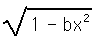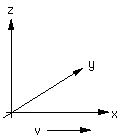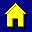Math is used extensively in physical science; it is difficult to present the concepts of relativity in much detail without it. Nevertheless, I will try to discuss as much of the material as possible without math, and add some equations (just algebra) to make the ideas clearer to those who are comfortable with the math.

Toward that end, I want to explain some things about the way math often has to be written online. Besides the familiar + and -, there are a few symbols that may be unfamiliar:

/   division
*   multiplication
^   power (exponent)
sqrt   square root

For example, x-squared would be written as x^2. The expression:would be written sqrt(1-b*x^2) or √(1-b*x^2).

Einstein's famous formulawould be E=mc^2.

Large numbers are written in scientific notation, using powers of 10. For instance, 100 is written as 102 or 1E+2, one billion is written as 109 or 1E+9.  Such notation is also useful in describing things such as the National Debt  ;-)

A few of more items of notation...

In physics, the symbol "c" denotes the speed of light. Velocity is v or u. The spatial coordinates are x, y, and z. For simplicity, we will always make reference frames move along the x-axis. The time coordinate is t.Return to the home page.